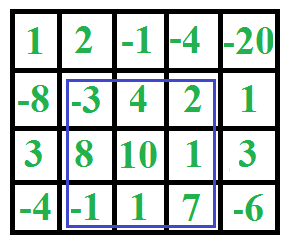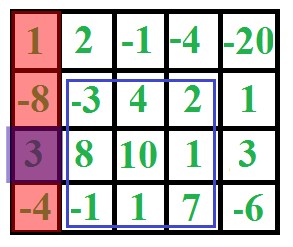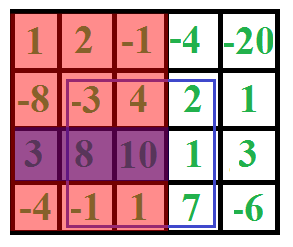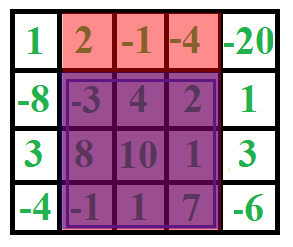## The Problem

Given an `M` x `N` matrix of integers, find the sub rectangle such that the sum is maximized.

## Example## Things to consider

• Negative values may appear in the matrix
• If all values were positive the maximum sum sub rectangle would be the entire matrix
• There is a better solution than checking every sub rectangle

## Data representation

• Nothing overly complicated needed
• A 2D array will represent the data exactly as needed
• Usually easier to use a vector<vector<T>> in C++ v.s. T[][] as passing 2D arrays with run time sizes into functions is a little awkward

## The Idea

• We can solve the one dimensional version of this problem in linear time using kadanes algorithm
• The rows of the 2D array can be flattened into a 1D array by summing them together
• We can then perform kadanes algorithm on the flattened rows and find the rows bounding the maximum sub sequence

## In Action• etc.## One Dimensional Algorithm

• In order to find the row bounds we need to implement the one dimensional maximum sub sequence algorithm
• Known as Kadane’s algorithm, it goes as follows:
• Keep track of the sum of the current prefix that we scanned
• If that prefix ever becomes negative, give up on it and start over
• After each iteration, compare our current prefix sum with the max seen so far and update accordingly

## Using this algorithm

• To use this algorithm, we simply need to iterate through the matrix fixing the columns
• We can then flatten the elements in between our fixed left and right columns into a one dimensional array
• Once we have our flattened columns, we run the result through kadanes algorithm and recieve the maximum sum as well as the bounding rows